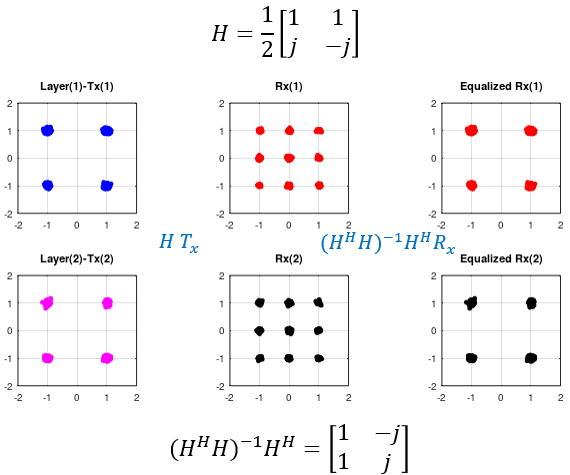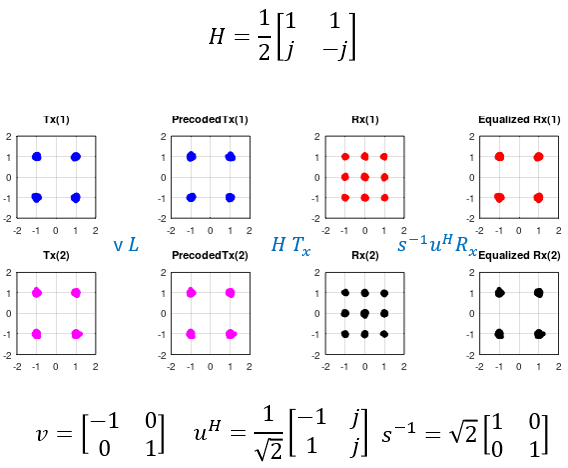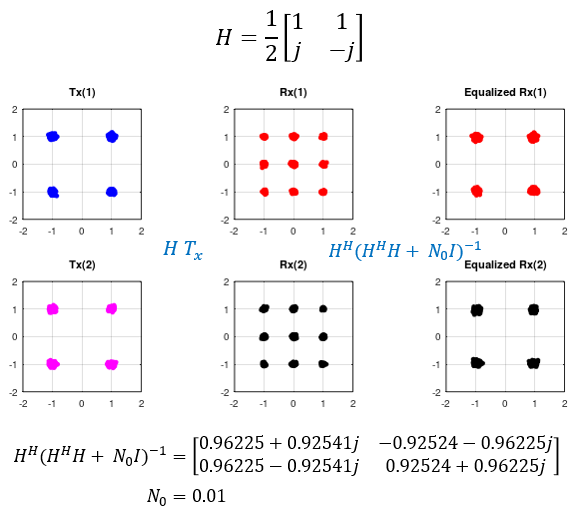Communication Technology What is Equalizer ?   In wireless communication (actually in any communication), the signal always go through a physical medium (mostly air and anything outside of the transmitter antenna in wirelss communication) before it reaches the reciever. In most cases, these channels tend not to be in the best environement for the signal and put some negative impact on the signal. As a result, the reciever would get the signal that is distorted or corrupted by the channel as illustrated below.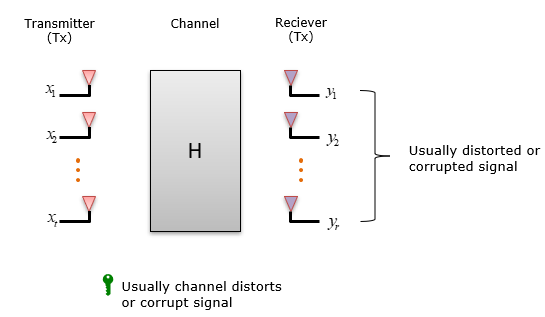If the distortion or corruption by the channel gets too serious, proper communication cannot happen. Then, how can we ensure that the transmitted signal(data) can be properly recieved(decoded) by the reciever even when the signal is distorted or corrupted by the wireless channel ? One most common solution is to use a special component called Equalizer. This component can be in the form of hardware or software or both. Equalizer is a special component(a block) that can UNDO the distortion / corruption done by channel while the signal pass through the channel.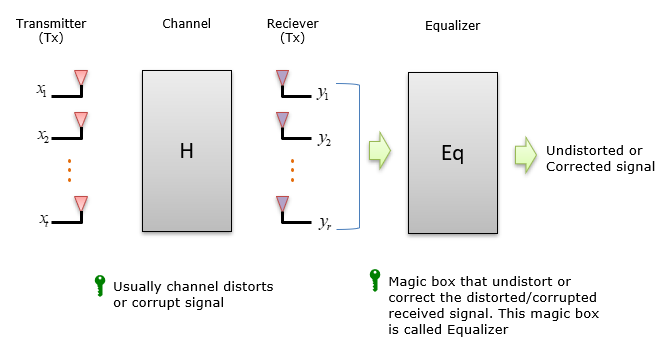How it works ?   It may sound like a magic when you first heard of what the equalizer do and may ask yourself how such a magic can happen. To explain on this, I would translate the block diagram shown in previous section into a mathematical format as shown below. (If you want to know about further details on H in this equation, refer to channel model page).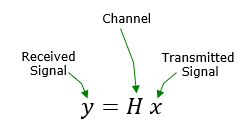As I mentioned above, usally the H distorts or corrupt the signal which make it difficult for the reciever to decode the signal. The goal is to undo the effect of H. Mathematical point of view, it is very simple. Just take the inverse of H (channel) and apply it to the received signal (Hx). Basically what Equalizer do is to calculate the inverse of channel matrix and correct (compensate) the received signal.Nothing is as easy as said   As everybody in the engineering would know, there is nothing as easy as said. Same applies to Equalization as well. As mentioned in previous section, ideally Equalization is just to take the inverse of channel matrix and applies it to the received signal, but there are some mathematical and technical chalenges and preconditions.   To obtain the inverse of the channel matrix, the channel matrix should satisfy specific conditions as follows. The channel matrix should be square matrix. It means that the number of Tx and Rx antenna should be same. But in reality, there are cases where Tx and Rx antenna are not same. The channel matrix should be invertable. It means the matrix should not be 0. 'A matrix is 0' mean 'it's determinant is 0'. To overcome these mathmatical restriction, we use many other techniques that can applies to non square matrix.  Most of the commonly used Equalization method like least squre, SVD, MMSE can be applied to non-square matrices.   Now let's suppose we have the means to overcome the mathematical constrains. But we still have some practical challenges to overcome.   Conceptually, Equalization is to get the inverse (or pseudo inverse) of the channel matrix. It mean that the channel matrix should be given to Equalizer. How do I (meaning the reciever) know about the channel matrix ? If channel does not change at all, we may investigate about the channel before we build the communication system and preprogram the channel matrix directly into the reciever, but we cannot expect this kind of constant (never changing) channel condition in wireless communication. Channel property changes all the time in wireless environment. It means the reciever itself has to figure out the channel characteristics and build the channel matrix dynamically. This process (i.e, finding out channel characteristics) is called channel estimation. There are various kind of channel estimation techniques and some of those techniques are explained in this page.       Examples     NOTE : Click on the image and it will lead you to the page with more examples and the Octave source code that I wrote for the examples.